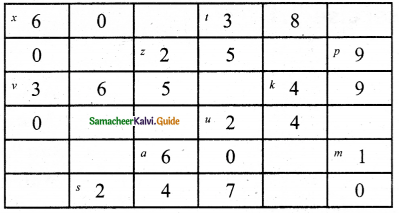Students can download Maths Chapter 2 Introduction to Algebra Ex 2.3 Questions and Answers, Notes, Samacheer Kalvi 6th Maths Guide Pdf helps you to revise the complete Tamilnadu State Board New Syllabus, helps students complete homework assignments and to score high marks in board exams.

## Tamilnadu Samacheer Kalvi 6th Maths Solutions Term 1 Chapter 2 Introduction to Algebra Ex 2.3

Miscellaneous Practice Problems

Question 1.
Complete the following pattern.
9 – 1 =
98 – 21 =
987 – 321 =
9876 – 4321 =
98765 – 54321 =
What comes next?
Solution:
9 – 1 = 8
98 – 21 = 77
987 – 321 = 666
9876 – 4321 = 5555
98765 – 54321 = 44444
Next will be 987654 – 654321 = 333333Question 2.
A piece of wire is ‘12s’ cm long. What will be the length of the side if it is formed as
(i) an equilateral triangle.
(ii) a square?
Solution:
(i) An equilateral triangle has 3 equal sides.
Length of the wire = ‘12s’ cm
Length of each side = $$\frac{12 s}{3}$$ cm.
Length of each side of the triangle = 4s cm
(ii) A square has four equal sides.
Length of each side $$\frac{12 s}{4}$$ cm
Length of each side of the square 3s cmQuestion 3.
Identify the value of the shapes and figures in the table given below and verify their addition horizontally and vertically.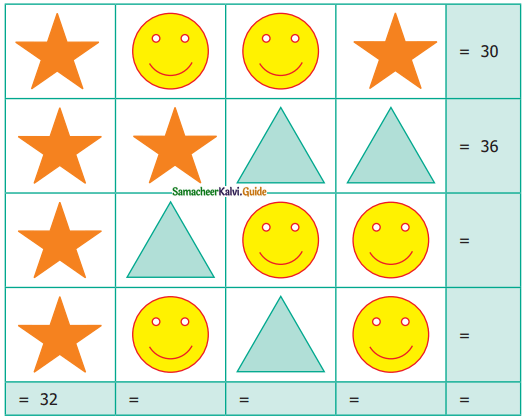Solution: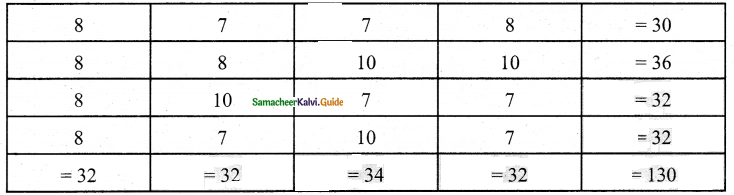Question 4.
The table given below shows the results of the matches played by 8 teams in a kabaddi championship tournament.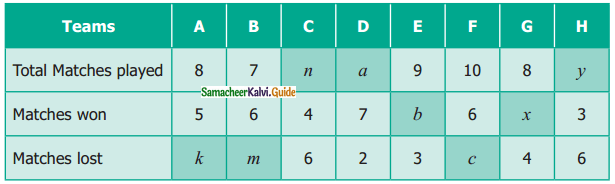Find the value of all the variables in the table given above.
Solution:
k = 3, m = 1, n = 10, a = 9, b = 6, c = 4, x = 4, y = 9Challenging Problems

Question 5.
Gopal is 8 years younger than Karnan. If the sum of their ages is 30, how old is Karnan?
Solution:
Let the age of Kaman be x years
Gopal’s age = x – 8
Ace to the problem, x + x – 8 = 30
2x – 8 = 30
2x = 30 + 8
2x = 38
x = $$\frac{38}{2}$$
x = 19
Age of Kaman = 19 yearsQuestion 6.
The rectangles made of identical square blocks with varying lengths but having only two square blocks as width are give below(i) How many small size squares are there in each of the rectangles P,Q, R and S?
(ii) Fill in the boxes.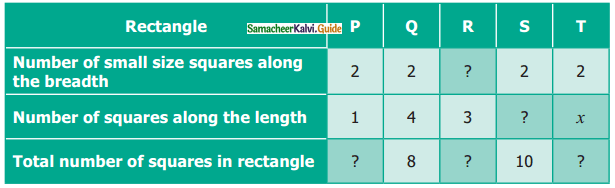Solution:
(i) P = 2; Q = 8; R = 6; S = 10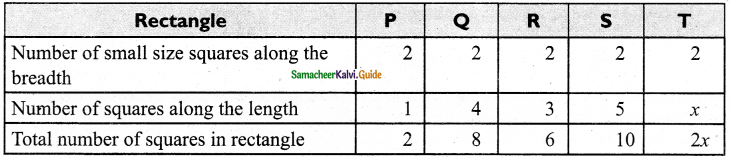Question 7.
Find the variables from the clues given below and solve the crossword puzzle.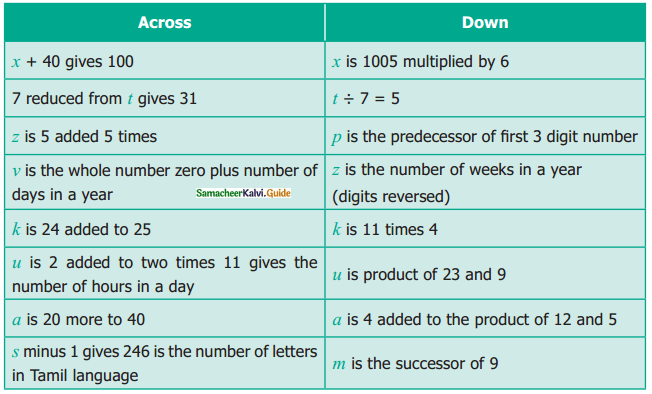Solution: